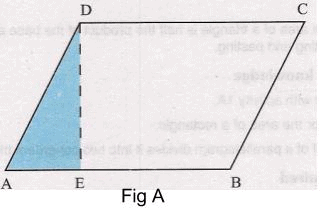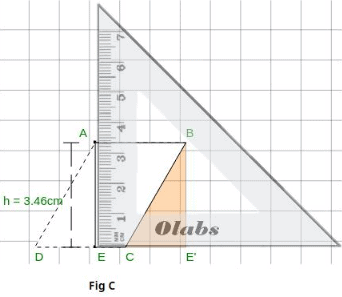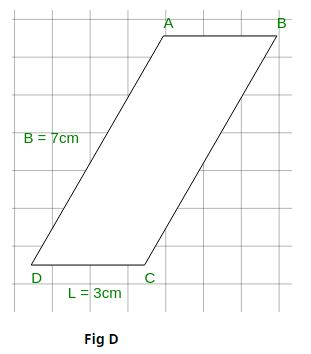# Procedure - To show that the Area of Parallelogram is Product of its Base and Height, Class 9 Math Class 9 Notes | EduRev

## Class 9 : Procedure - To show that the Area of Parallelogram is Product of its Base and Height, Class 9 Math Class 9 Notes | EduRev

The document Procedure - To show that the Area of Parallelogram is Product of its Base and Height, Class 9 Math Class 9 Notes | EduRev is a part of the Class 9 Course Mathematics (Maths) Class 9.
All you need of Class 9 at this link: Class 9

As performed in the real lab:

Materials Required:

Glazed paper, pencil, a pair of scissors, gum.

Procedure:

1. Make a parallelogram by paper folding. Call it ABCD.
2. Cut out the parallelogram with the help of a pair of scissors.
3. Obtain a perpendicular from D to AB meeting AB at E. [Fig A]
4. Cut and remove the triangle AED and align AD with BC. Call the displaced segment AE as BE'. [Fig B]
5. Verify using a scale that EBE' are collinear.
6. Verify that CE' is perpendicular to EBE and EE' = CD
7. Observe that the figure obtained is a rectangle.[Fig B]As performed in the simulator:

1. Create a parallelogram ABCD with length L and breadth B.[Fig C]

2. Draw perpendicular from A to DC meeting at point E.

1.     Click on "Set Square" in Tools to use it.

2.     Drag and place Set Square such that point A and line DC is perpendicular.

3. Click on ▲ AED to separate it from parallelogram.

4. Drag ▲ AED and place it such a way that AD is overlapped with BC.Observation:

1. E is Co-linear with base.
2. Line DE is perpendicular to base.
3. Therefore it will forms rectangle ABE'E.
4. Thus the area of parallelogram = area of rectangle ABE'E

Note:

In some input cases, perpendicular of parallelogram may fall outside the base [E.g. Fig D]. In such cases click on parallelogram to rotate it and follow the same procedure as mentioned above.Result:

Area of parallelogram is the product of its base and height.

Offer running on EduRev: Apply code STAYHOME200 to get INR 200 off on our premium plan EduRev Infinity!

## Mathematics (Maths) Class 9

190 videos|233 docs|82 tests

,

,

,

,

,

,

,

,

,

,

,

,

,

,

,

,

,

,

,

,

,

,

,

,

;